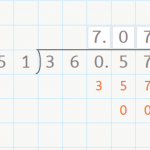Smartick is an advanced online program that teaches kids math and coding in only 15 min. a day

# I want to learn about: Decimals

Learn about decimal numbers with Smartick. Exercises, tutorials problems, and teaching resources about decimal numbers for elementary aged children. Here you will find posts about decimal numbers to make learning mathematics easier and more fun.

Jun10

## Dividing a Decimal by a Whole NumberDividing a Decimal by a Whole Number Hello everyone! In this week’s post, we are going to talk about dividing a decimal by a whole number. You already know how to divide a whole number by a whole number by now, right? If not, check out our posts about dividing by a one-digit number here, […]